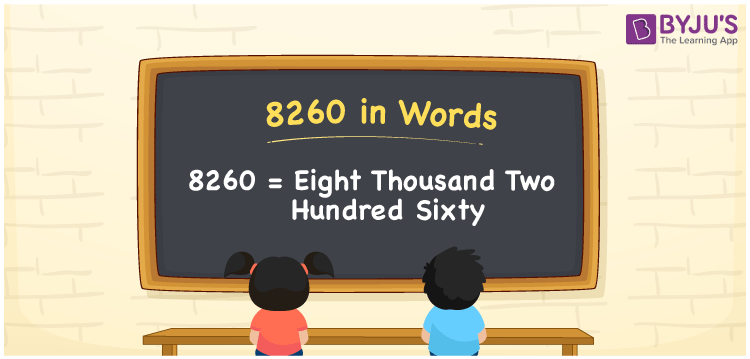# 8260 in Words

8260 in words is written as Eight thousand two hundred sixty. In both the International System of Numerals and the Indian System of Numerals, 8260 is written as Eight thousand two hundred sixty. The number 8260 is a Cardinal Number as it represents some quantity. For example, “the dress costs 8260 rupees.”

 8260 in Words Eight thousand two hundred sixty Eight thousand two hundred sixty in Number 8260

## 8260 in English Words

8260 in English words is read as “Eight thousand two hundred sixty.”## How to Write 8260 in Words?

To write 8260 in words, we shall use the place value chart. In the place value chart, put 8 in the thousands, 2 in the hundreds, 6 in the tens and 0 in the ones, respectively. Let us make a place value chart to write the number 8260 in words.

 Thousands Hundreds Tens Ones 8 2 6 0

Thus, we can write the expanded form as

8 × Thousand + 2 × Hundred + 6 × Ten + 0 × One

= 8 × 1000 + 2 × 100 + 6 × 10 + 0 × 1

= 8000 + 200 + 60 + 0

= 8260

= Eight thousand two hundred sixty.

8260 is a natural number, the successor of 8259 and the predecessor of 8261.

8260 in words – Eight thousand two hundred sixty

• Is 8260 an odd number? – No
• Is 8260 an even number? – Yes
• Is 8260 a perfect square number? – No
• Is 8260 a perfect cube number? – No
• Is 8260 a prime number? – No
• Is 8260 a composite number? – Yes

## Frequently Asked Questions on 8260 in Words

Q1

### How to write 8260 in words?

8260 in words is written as Eight thousand two hundred sixty.
Q2

### How to write 8260 in words in the International and Indian System of Numerals?

In both, the system of numerals, 8260 in words, is written as Eight thousand two hundred sixty.
Q3

### How to write 8260 in a place value chart?

In the place value chart, write 8 in the thousands, 2 in the hundreds, 6 in the tens and 0 in the ones, respectively.